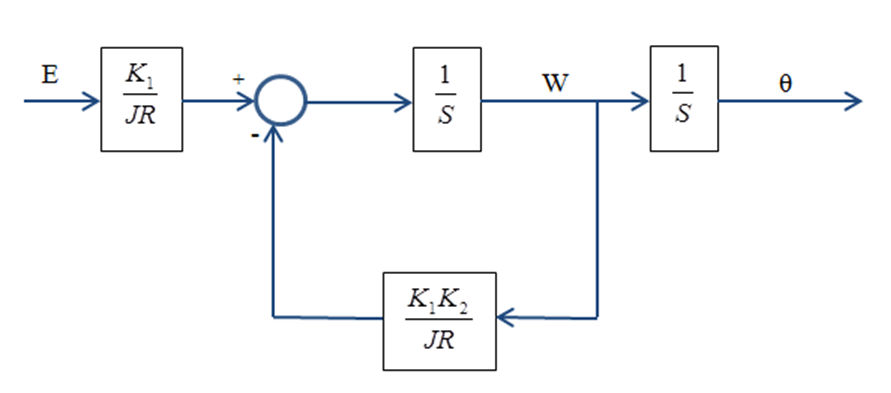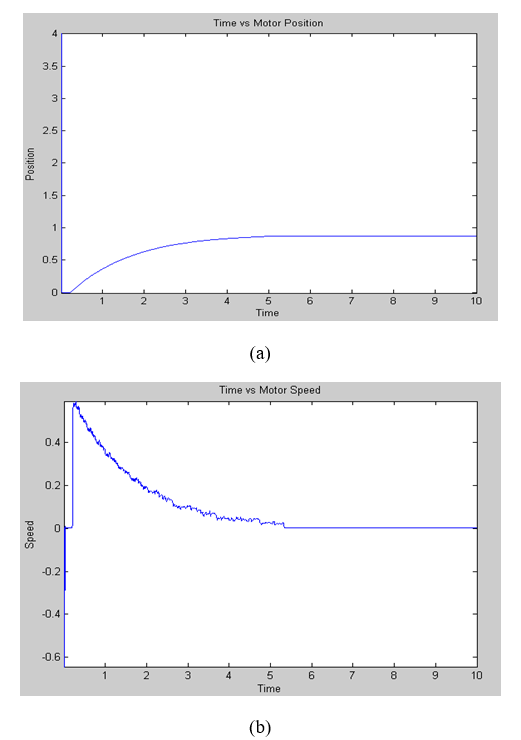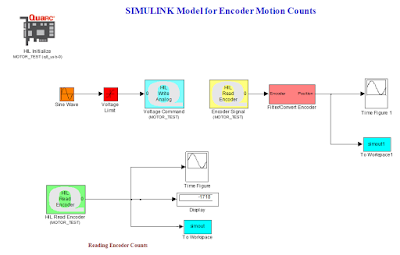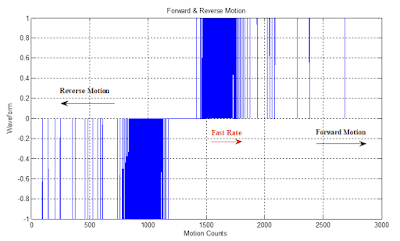## Welcome to the World of Modelling and Simulation

### What is Modelling?

This blog is all about system dynamics modelling and simulation applied in the engineering field, especially mechanical, electrical, and ...### Suppression of Rubbing in a Rotating Machine by a Novel Lemon Shaped Bea...

This video shows an experiment on how to minimize rubbing between a rotor and a guide that typically happens in a high speed rotating machine with a newly developed lemon shaped bearing or guide. Although, it might not seem relevant to the context of this blog; but it is here for the visualization purpose of the interaction of real components in a typical rotating machine. We see here as the speed goes high, the system enters into its resonance frequency where it vibrates excessively. The lemon shaped bearing or guide helps prevent the excessive rubbing while the rotor-bearing assembly is in the natural frequency zone. It has been found from our research that lemon shaped guide minimizes rubbing better than the circular guide (https://doi.org/10.1115/1.4043817). It should be noted that experiment is the best way of visualization; although, it is sometimes difficult to conduct an experiment due to cost, lack of materials, facilities and so on. In this case, modelling-simulation is alternatively the better way for visualization.

### Suppression of Rubbing in a Rotor-Stator Assembly by Circular Bearing/Guide

This video shows an experiment on how to minimize rubbing between a rotor and a guide that typically happens in a high speed rotating machine with a circular bearing or guide. Although, it might not seem relevant to the context of this blog; but it is here for the visualization purpose of the interaction of real components in a typical rotating machine. We see here as the speed goes high, the system enters into its resonance frequency where it vibrates excessively. The circular bearing or guide helps prevent the excessive rubbing while the rotor-bearing assembly is in the natural frequency zone. It should be noted that experiment is the best way of visualization; although, it is sometimes difficult to conduct an experiment due to cost, lack of materials, facilities and so on. In this case, modelling-simulation is alternatively the better way for visualization.

### Discussion on Linear Quadratic Optimal Control

Linear quadratic optimal control is popular in industry and is simple, yet powerful tool to achieve the desired controller design. In this study, the model of the plant is the familiar DC motor. This report concerns systematic approach to develop the linear quadratic optimal controller. To do this, first the mathematical model of the DC motor is developed, then the development of the LQR controller is described and finally an experiment is conducted to gain in-depth knowledge in controller design.

1. DC Motor State-Space Model Development 
DC motor converts electrical energy (input voltage) to mechanical energy (shaft rotation). This electromechanical conversion involves Faraday’s law of induction and Ampere’s law for force generated on the conductor moving in a magnetic field. In ideal situation, the torque (T) developed on the motor shaft is proportional to the input current (I) and the induced electromotive force (EMF) (V) or back EMF is proportional to the speed (W) of the motor. This can be expressed as;

Where, K1 and K2 are the proportionality constant.
The electrical power (Pe) input to the motor is the product of the induced EMF and current.

And the mechanical power output (Pm) is the product of the speed of the motor and torque.

From Ohm’s law, it is known that,

Where, E is the input voltage to the motor and R is the resistance of the motor armature.
Moreover, we also know that torque produced at the motor shaft is equal to the product of the inertia of the load (J) and rate of change of angular velocity or angular acceleration.

Now, from equations (1), (6) and (7), it is found that

Using equation (2) further, the following expression can be established.

The above equation refers to the first order linear differential equation model where ‘W’ represents the state of the system and ‘E’ is the external control input. This first order equation is good enough to predict the output speed of the motor. However, in terms of measuring the position’ it is necessary to add the following equation.

Where θ is the output position of the DC motor and refers to another state of the system. Therefore, the model has one control input and two state variables (position and velocity).
Now, from equations (9) and (10), the state space model can be formulated.

Equation (11) is the model representation in state space form.
Figure (1) highlights the block diagram of the DC motor dynamic model.
From equation (9), after rearranging the terms

The coefficients in the equation (12) may be assumed as the following two constants.

Where ‘τ’ refers the time constant and ‘c’ is the DC gain.Figure 1: Block diagram of the system represents the dynamics of the DC motor driving inertial load.

So, equation (12) can be written in simplified form with time constant and DC gain.

The values of τ and c will be determined by the experiment. The SIMULINK “DC MOTOR MODEL” along with the physical setup in the EEEL 155 lab has been used. The time constant and DC gain of the motor are measured in response to a unit step input.
From the experiment, the open loop response of the DC motor is shown in figure 2.
It is found that the time constant is 0.03s and c is 1.65 rad/s/volt.
Substitution of the c and τ values in equation (11) forms the matrices of the state space below.

The calculation for the constants in the above matrices is shown below.

2. LQR Design Method
To design the controller, MATLAB is used rigorously. The first step in the designing process is to check the rank of the controllability matrix. And, this could easily be done by using MATLAB commands ‘ctrb’ and ‘rank’. 'ctrb' is used to generate the controllability matrix and then 'rank' gives the rank of the generated matrix .

Figure 2: Open loop response of the DC motor to the unit step input.

2.1 Rank of Controllability Matrix Checking:

>> A=[0 1; 0 -33.33];
>> B=[0; 55];
>> C=[1 0; 0 1];
>> D=;
>> M=ctrb(A,B);
>> N=rank(M)
N =
2

As the state transition matrix is 2x2 and the rank of the controllability matrix shows 2, therefore our system is state controllable.

From the state space model, following A, B, C and D matrices are obtained below.

Now, the linear quadratic optimal controller (LQR) development is shown in the following section.

2.2 System Open loop Transfer Functions:
Equation (13) is shown here again to determine the open loop transfer function of the second order system.

Taking Laplace transform on both sides of the above equation;

And, by considering speed as the output, the open loop transfer function of the first order system is,

Taking Laplace transformation,

2.3 System Stability Checking:
To check the stability of the system, MATLAB commands ‘poly’ and ‘roots’ are implemented. ‘poly’ generates the coefficients of the characteristic equation and ‘roots’ then determines the eigenvalues of the A matrix .

>> poly(A)
ans =
1.0000   33.3300         0
>> roots(poly(A))
ans =
0
-33.3300

As there is no pole on the right side of the s-plane, so the system is stable. Due to not considering the damping, a pole just lies at the origin in the s-plane which further reminds the marginally stable situation.

2.4 Model Validation by Experiment:
The mathematical model of the DC motor system developed before has to be validated. First the open loop system response to the unit step input will be observed by the model and then by experiment.Figure 3: Open loop response of the DC motor model to the unit step input.

From figure 3, it is clear that DC gain is 1.65 rad/s/v and time constant is measured 0.03s. And, these values are exactly same to the experimental values specified in figure 2. The MATLAB m-file program to represent the mathematical model along with output plots is shown below.

2.5 Coding to Generate the First Order DC Motor Model Response

close all;
clear all;
format long;
more off;

A=[0 1; 0 -33.33];

B=[0; 55];
C=[1 0; 0 1];
D=0;

states = {'x' 'x_dot'};

inputs = {'r'};
outputs = {'x'; 'x_dot'};

sys_ss = ss(A,B,C,D,'statename',states,'inputname',inputs,'outputname',outputs);

t=0:0.001:5;

r=ones(size(t));
[y,t]=lsim(sys_ss,r,t);

figure(1)

plot(t,y(:,1))
title('DC Motor Response To Unit Step')
xlabel('Time')
ylabel('Angular Displacement of DC Motor')

figure(2)

plot(t,y(:,2))
title('DC Motor Response To Unit Step')
xlabel('Time')
ylabel('Angular Velocity of DC Motor')

2.6 Designing the Controller:
Designing a controller predominantly depends on the demand or criteria for the specific case. A controller may be designed for a given purpose and the same controller may further be implemented in different way in another application. So, in this study, a very general and simple control problem is defined. The problem may be described as follows;
When LQR is implemented for system control, the system eventually becomes closed loop and a state feedback gain matrix ‘K’ is generated by the controller which determines the control strategy based on the weights on the states and control effort. For this DC motor problem, there are two states: position and velocity. Input is step which is generated by computer. A program is written in MATLAB m-file for designing the LQR controller. The cost function is the important thing which places relative importance between the states and input. When designing the controller, first the weights on the states are considered 1 unit and weight on actuation is taken 1 unit also. And, this creates a problem which is shown by the following figures.Figure 4: Close loop response of the DC motor model when LQR is implemented by MATLAB (a) Position and (b) Speed.

From figure 4, the position and speed are not at all desirable as the position should increase linearly with the increase of time and the speed was found from experiment (fig. 2) and simulation (fig. 3) also contradicts the result. So, the objective here is to bring back the motor speed and position as before by tuning the LQR controller which is to optimize the relative importance between states and inputs. Figure 5 shows the results from the experiment which are quite conforming to the simulation results.Figure 5: DC motor response when LQR is implemented in experiment (a) Position and (b) Speed.

Therefore, this section may be divided into two sub-sections for the controller design aspects:
Designing LQR controller in MATLAB environment
Validating the simulation by experiment qualitatively

A MATLAB function 'lqr ()' provided in the Control Systems Toolbox can be used to design an LQR for a given system with specified weighting matrices. The syntax of the function may be written as [K,P]=lqr(A,B,Q,R) , where (A,B) is the given state space model, and Q and R are the weighting matrices. Q weights on states and R weights on control. K is the state feedback gain matrix, and P is the solution matrix for the algebraic Riccati equation (ARE) .
A Program is written in MATLAB m-file to solve the ordinary problem as mentioned before. Controlling the states (position & velocity) is 1000000 times more important than the actuation. Considering this fact in cost function, the following program explains the steps of the LQR implementation and control achievement.

2.6.1.1. MATLAB Program for DC Motor Control by LQR:

close all;
clear all;
format long;
more off;
A=[0 1; 0 -33.33];                % Model of the DC Motor in State Space
B=[0; 55];
C=[1 0; 0 1];
D=[0; 0];
Q=[0.000001 0; 0 0.000001]'*[0.000001 0; 0 0.000001];
R=[0; 1]'*[0; 1];
K=lqr(A,B,Q,R);                   % Implementation of LQR Controller
Ac=(A-B*K);                       % New System Matrices by State Feedback Gain
Bc=B;
Cc=C;
Dc=D;
states={'x' 'x_dot'};
inputs={'r'};
outputs={'x'; 'x_dot'};
sys_cl = ss(Ac,Bc,Cc,Dc,'statename',states,'inputname',inputs,'outputname',outputs);
t=0:0.001:10;                     % Simulation Period
r=ones(size(t));
[y,t]=lsim(sys_cl,r,t);           % System Response to Step Input
figure(1)
plot(t,y(:,1))
title('Step Response with LQR Control')
xlabel('Time')
ylabel('Angular Displacement of DC Motor')
figure(2)
plot(t,y(:,2))
title('Step Response with LQR Control')
xlabel('Time')
ylabel('Angular Velocity of DC Motor')Figure 6: Closed loop response of the DC motor model when cost function of LQR is changed from before (fig.4) (a) Position and (b) Speed.

From figure 6, it is evident that the motor response shows its original response. And, this confirms the success of the controller design by simulation. In the next section, the simulation results will further be verified by the experiment.

2.6.2 Experiment:
A SIMULINK model is developed to see the effects of the weights on the states of the DC motor. Figure 7 shows the model. This model is a real time simulation which is eventually possible by QUARC toolbox in SIMULINK. From fig.7, there are two feedback paths for the motor speed and position which are measured by the sensors (tachometer & encoder). There is no dedicated SIMULINK block for the LQR application in the MATLAB version of EEEL 155 computers. So, two feedback gains are introduced in the model for the corresponding states. And, the weights on the states are kept same as before (m-file program) for understanding optimal control policy by LQR and validation of the predictions in simulations.Figure 7: SIMULINK model to study the influence and validation of weights increment of the states. Figure 8 is obtained from the experiment. The position and speed show the promising effects of relative weights on the states which are quite similar to the predictions in figure 6.Figure 8: DC motor response when modified LQR is implemented in experiment (a) Position and (b) Speed.

References
  Control system design : an introduction to state-space methods / Bernard Freidland.—Dover ed.  Ogata, K., 2002, Modern Control Engineering, Prentice Hall, Upper Saddle River, NJ.  Linear feedback control: analysis and design with MATLAB / Dingyü Xue, YangQuan Chen, Derek P. Atherton., the Society for Industrial and Applied Mathematics, 2007.#Blog #Blogger #LQR #Matlab #Simulink #ControlSystemDesign #StateSpace

### A SIMULINK Model for Understanding Encoding and Decoding

Encoders and Decoders

Optical encoders can be classified in two ways:
• Absolute Encoder
• Relative/Incremental Encoder
Encoders may have linear or rotary configurations. However, the rotary encoders are common in industry. In absolute encoder, a unique digital word corresponds to each rotational position of the shaft whereas in incremental encoder, by producing digital pulses while the shaft rotates measure the relative displacement of the shaft .
In this lab, Incremental encoder is studied and implemented. Incremental encoders are simple in construction and measurement as well.

Objectives
1. To develop a MATLAB program which decodes the input data streams “idatal8” given in the lab materials.
2. Then translating the written MATLAB code into a SIMULINK program.
3. Verification of the decoders by experiment comparing with the built-in decoders in the DC motor kit.

Objective 1
A MATLAB program is developed to decode the input data file “idatal8”. The steps and explanation of the commands are given as notes to the respective lines in the program. The entire program is given below which is based on the decoding logic given in table 1:

Table 1: Decoding of Optical Encoder Signal for Position Measurement 
 Trigger Other Channel Motion Count A rising (0 to 1) B high (1) -1 A rising (0 to 1) B low (0) +1 B rising (0 to 1) A high (1) +1 B rising (0 to 1) A low (0) -1 A falling (1 to 0) B high (1) +1 A falling (1 to 0) B low (0) -1 B falling (1 to 0) A high (1) -1 B falling (1 to 0) A low (0) +1

MATLAB Program for Decoding Input Data Streams

close all;

clear all;
format long;

idatal8                         % Running the input data streams

t=1:179;                        % Total Sample

A=digdata(1,1:179);             % Splitting the "digdata" into two workspaces
B=digdata(2,1:179);

j=1;

for i=1:178;                    % logic created for decoding input data

sum=0;

p=A(1,j);
q=B(1,j);
r=A(1,j+1);
s=B(1,j+1);

if r>p && q==0          % A rising & B low

count=1;

elseif p==1 && s>q      % A high & B rising

count=1;

elseif r<p && q==1      % A falling & B high

count=1;

elseif p==0 && s<q      % A low & B falling

count=1;

elseif p==0 && s>q      % A low & B rising

count=-1;

elseif r>p && q==1      % A rising & B high

count=-1;

elseif p==1 && s<q      % A high & B falling

count=-1;

elseif r<p && q==0      % A falling & B low

count=-1;

else

count=0;

end

sum=sum+count;

X(1,j)=sum;             % Representing motion after decoding

j=j+1;

end

figure(1)

subplot (211),plot(t,A,'b'),grid
title('Sequence of Pulses vs Quadrature Signals')
ylabel ('Waveform from Channel A'),xlabel('Pulse Sequences')
subplot (212),plot(t,B,'r'),grid
title('Sequence of Pulses vs Quadrature Signals')
ylabel ('Waveform from Channel B'),xlabel('Pulse Sequences')

figure(2)                       % Decoded Motion

t1=1:178;
plot(t1,X,'b'),grid
title('Forward & Reverse Motion')
ylabel ('Waveform'),xlabel('Motion Counts')

Following figure 1 shows the waveform from channel A and B plotted separately obtained from the data file ‘idatal8’. Figure 2 represents the motion count after decoding the input data streams which eventually dictate the forward and reverse direction by the program algorithm. This figure also highlights the fast and slow sequences of the counts.Figure 1: Showing the signals from channel A and B from input data streams ‘idatal8’.Figure 2: Showing the decoded signal from input data streams ‘idatal8’ representing motion.

Objective 2
An incremental or quadrature encoder provides two bit signal. Each signal comes from each of the receivers (channel A and B). These two input streams are offset by ¼ of one of the light or dark bands. For an incremental encoder, the will be four discrete positions for each pulse. These positions are (0,0), (1,0), (1,1) and (0,1). Depending on these 4 distinct positions, the direction of rotation of the motor may be determined by the following table.

Table 2: Direction of Motion Assessment

 Forward Motion Channel A 0          1          1          0          0          1          1          0................... Channel B 0          0          1          1          0          0          1          1................... Backward Motion Channel A 1          0          0          1          1          0          0          1.................. Channel B 0          0          1          1          0          0          1          1..................

A SIMULINK model is created to understanding the position and velocity counting mechanisms of the encoder which is connected to the “DC Motor Kit”. Following figure 3 shows the simple model for the experiment. In the experiment, attempts are taken to measure the resolution of the physical encoder. For this, first the total number of discrete positions is counted for one revolution. For one revolution, there are 7820 distinct points and number of bands or slots on the disk is 1955 (7820/4). For one revolution, the encoder count decreases from 0 to -3950 and then increases to -80 and based on this information the 7820 discrete points are estimated. This calculation might vary, but would provide some insights into position measurement. So, the resolution of the experimental encoder is 0.04º (360/7820) approximately. For each positive or negative counts, there will be a change of position of the motor around 0.04º that the encoder measures.Figure 3: A SIMULINK model for understanding encoding and decoding.

Objective 3
In this part, the algorithm developed earlier in Objective-1 will be verified. For this, the encoder total counts for a single rotation is gathered and stored in the workspace. A truncated data table is provided below to show the trend of the encoder counts.

Table 3: Encoder Reading Pattern from the Experiment

 -1469 -1467 -1465 -1463 -1461 -1459 -1457 -1455 -1453 -1451 Data Truncated -1701 -1703 -1705 -1707 -1709 -1711 -1713 -1715 -1716 -1718

To verify the MATLAB program, the similar program with some modifications is done which decodes the above data streams. The program for the physical encoder decoding is given below:

MATLAB Program for Decoding Physical Encoder Counts

close all;
clear all;
format long;

inputdatastreams                        % Running data file

t=(1:2707)';
A=counts;

j=1;
for i=1:2707;
sum=0;
p=A(j,1);
q=A(j+1,1);
if q>p                          % Decoding logics
count=-1;
elseif q<p
count=1;
else
count=0;
end
sum=sum+count;
X(j,1)=sum;                     % Stores positive/negative counts

j=j+1;
end

figure(1)

plot(t,X,'b'),grid
title('Forward & Reverse Motion')
ylabel ('Waveform'),xlabel('Motion Counts')

Figure 4 shows the decoded signals from the encoder. From the figure, it is observed that the data streams have forward motion, reverse motion, fast and slow rate with the increase of time.Figure 4: Showing the decoded signal from encoder data streams.

References
 David G. Alciatore, Michael B. Histand, Introduction to Mechatronics and Measurement Systems-fourth edition, The McGraw-Hill Companies, Inc., 2012.
 Dr. Jeff Pieper, ENME 560 (Mechatronics Design Laboratory), Lab-05 Manual, 2006.

#Encoder #Decoder #IncrementalEncoder #DataStream #SIMULINK #Blog #Blogger